# imlpt

Inverse multiscale local 1-D polynomial transform

## Syntax

``y = imlpt(coefs,T,coefsPerLevel,scalingMoments)``
``y = imlpt(___,Name,Value)``

## Description

example

````y = imlpt(coefs,T,coefsPerLevel,scalingMoments)` returns the inverse multiscale local polynomial 1-D transform (MLPT) of `coefs`. The inputs to `imlpt` must be the outputs of `mlpt`.```

example

````y = imlpt(___,Name,Value)` specifies `mlpt` properties using one or more `Name,Value` pair arguments and the input arguments from the previous syntax.```

## Examples

collapse all

Create a signal with nonuniform sampling and verify good reconstruction when performing the `mlpt` and `imlpt`.

Create and plot a sine wave with non-uniform sampling.

```timeVector = 0:0.01:1; sineWave = sin(2*pi*timeVector)'; samplesToErase = randi(100,100,1); sineWave(samplesToErase) = []; timeVector(samplesToErase) = []; figure(1) plot(timeVector,sineWave,'o') hold on```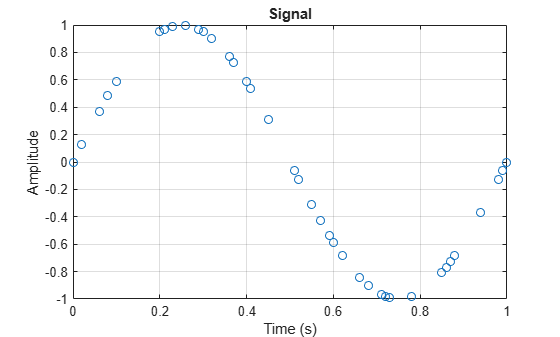Perform the multiscale local 1-D polynomial transform (`mlpt`) on the signal. Visualize the coefficients.

```[coefs,T,coefsPerLevel,scalingMoments] = mlpt(sineWave,timeVector); figure(2) stem(coefs) title('Wavelet Coefficients')```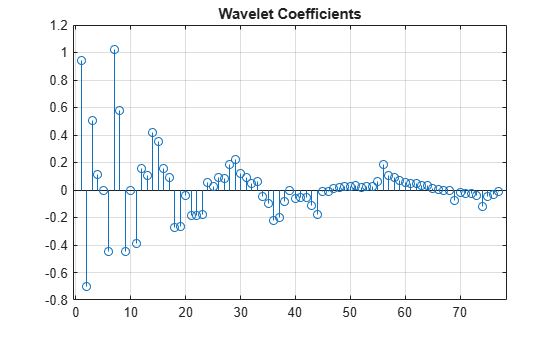Perform the inverse multiscale local 1-D polynomial transform (`imlpt`) on the coefficients. Visualize the reconstructed signal.

```y = imlpt(coefs,T,coefsPerLevel,scalingMoments); figure(1) plot(T,y,'*') legend('Original Signal','Reconstructed Signal') hold off```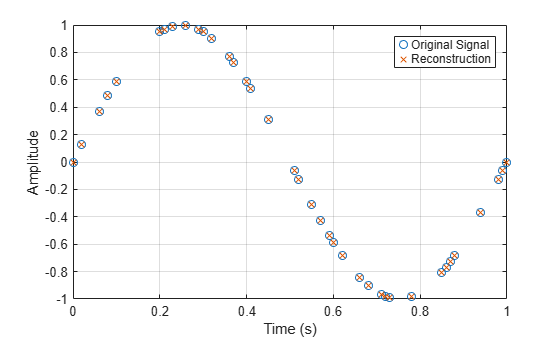Look at the total error to verify good reconstruction.

`reconstructionError = sum(abs(y-sineWave))`
```reconstructionError = 2.8383e-15 ```

Specify nondefault dual moments by using the `mlpt` function. Compare the results of analysis and synthesis using the default and nondefault dual moments.

Create an input signal and visualize it.

```T = (1:16)'; x = T.^2; plot(x) hold on```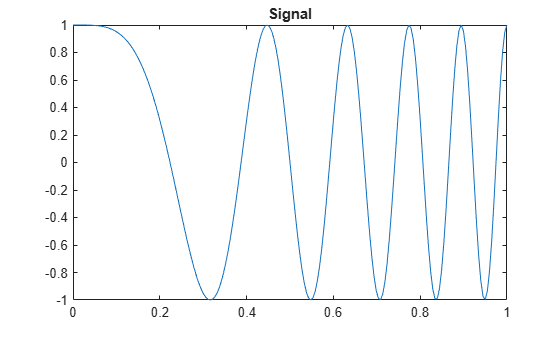Perform the forward and inverse transform for the input signal using the default and nondefault dual moments.

```[w2,t2,nj2,scalingmoments2] = mlpt(x,T); y2 = imlpt(w2,t2,nj2,scalingmoments2); [w3,t3,nj3,scalingmoments3] = mlpt(x,T,'dualmoments',3); y3 = imlpt(w3,t3,nj3,scalingmoments3,'dualmoments',3);```

Plot the reconstructed signal and verify perfect reconstruction using both the default and nondefault dual moments.

```plot(y2,'o') plot(y3,'*') legend('Original Signal', ... 'DualMoments = 3', ... 'DualMoments = 2 (Default)'); fprintf('\nMean Reconstruction Error:\n');```
```Mean Reconstruction Error: ```
`fprintf(' - Nondefault dual moments: %0.2f\n',mean(abs(y3-x)));`
``` - Nondefault dual moments: 0.00 ```
`fprintf(' - Default dual moments: %0.2f\n\n',mean(abs(y2-x)));`
``` - Default dual moments: 0.00 ```
`hold off`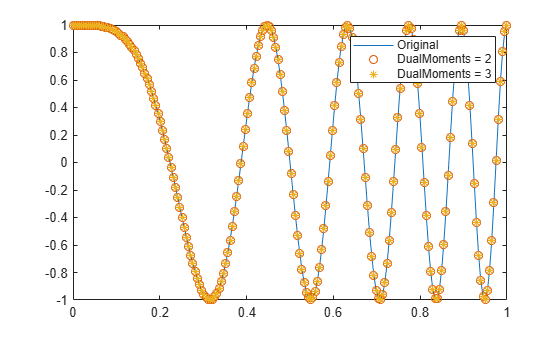## Input Arguments

collapse all

MLPT coefficients, specified as a vector or matrix of MLPT coefficients returned by the `mlpt` function.

Data Types: `double`

Sampling instants corresponding to `y`, specified as a vector or `duration` array of increasing values returned by the `mlpt` function.

Data Types: `double` | `duration`

Coefficients per resolution level, specified as a vector containing the number of coefficients at each resolution level in `coefs`. `coefsPerLevel` is an output argument of the `mlpt` function.

The elements of `coefsPerLevel` are organized as follows:

• `coefsPerLevel(1)` — Number of approximation coefficients at the coarsest resolution level.

• `coefsPerLevel(i)` — Number of detail coefficients at resolution level `i`, where ```i = numLevel – i + 2``` for ```i = 2, ..., numLevel + 1```. `numLevel` is the number of resolution levels used to calculate the MLPT. `numLevel` is inferred from `coefsPerLevel`: ```numLevel = length(coefsPerLevel-1)```.

The smaller the index `i`, the lower the resolution. The MLPT is two times redundant in the number of detail coefficients, but not in the number of approximation coefficients.

Data Types: `double`

Scaling function moments, specified as a `length(coefs)`-by-`P` matrix, where `P` is the number of primal moments specified by the MLPT.

Data Types: `double`

### Name-Value Arguments

Specify optional comma-separated pairs of `Name,Value` arguments. `Name` is the argument name and `Value` is the corresponding value. `Name` must appear inside quotes. You can specify several name and value pair arguments in any order as `Name1,Value1,...,NameN,ValueN`.

Example: `'DualMoments',3` computes a transform using three dual vanishing moments.

Number of dual vanishing moments in the lifting scheme, specified as the comma-separated pair consisting of `'DualMoments'` and `2`, `3` or `4`. The number of dual moments must match the number used by `mlpt`.

Data Types: `double`

## Output Arguments

collapse all

Reconstructed signal, returned as a vector or matrix, depending on the inputs to the `mlpt` function.

Data Types: `double`

## Algorithms

Maarten Jansen developed the theoretical foundation of the multiscale local polynomial transform (MLPT) and algorithms for its efficient computation . The MLPT uses a lifting scheme, wherein a kernel function smooths fine-scale coefficients with a given bandwidth to obtain the coarser resolution coefficients. The `mlpt` function uses only local polynomial interpolation, but the technique developed by Jansen is more general and admits many other kernel types with adjustable bandwidths .

 Jansen, Maarten. “Multiscale Local Polynomial Smoothing in a Lifted Pyramid for Non-Equispaced Data.” IEEE Transactions on Signal Processing 61, no. 3 (February 2013): 545–55. https://doi.org/10.1109/TSP.2012.2225059.

 Jansen, Maarten, and Mohamed Amghar. “Multiscale Local Polynomial Decompositions Using Bandwidths as Scales.” Statistics and Computing 27, no. 5 (September 2017): 1383–99. https://doi.org/10.1007/s11222-016-9692-8.

 Jansen, Maarten, and Patrick Oonincx. Second Generation Wavelets and Applications. London ; New York: Springer, 2005.

## SupportGet trial now